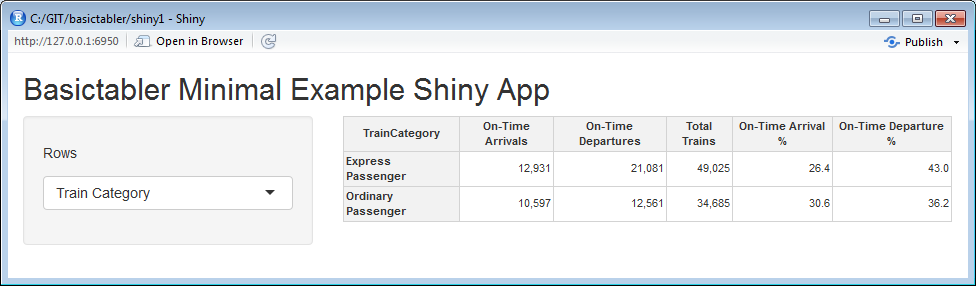## In This Vignette

• Examples
• Minimal Example

Shiny is an R package that makes it easy to build interactive web applications (apps) straight from R.

Good starting points for using Shiny are:

## Examples

This vignette presents a basic example to get started using basictabler with Shiny. It is not intended to be a fully featured application, rather to help the reader get started quickly using basictabler with Shiny.

To use the example, create a new single-file Shiny web application in RStudio, then paste the R code into the app.R file.

## Minimal Example

library(shiny)
library(htmlwidgets)
library(dplyr)
library(basictabler)

ui <- fluidPage(

titlePanel("Basictabler Minimal Example Shiny App"),

sidebarLayout(
sidebarPanel(

selectInput("groupBy", label = h5("Rows"),
choices = list("Train Category" = "TrainCategory",
"TOC" = "TOC",
"Power Type" = "PowerType"), selected = "TOC")
),

mainPanel(
basictablerOutput('tbl')
)
)
)

server <- function(input, output) {

output$tbl <- renderBasictabler({ tocsummary <- bhmsummary %>% group_by_(input$groupBy) %>%
summarise(OnTimeArrivals=sum(OnTimeArrivals),
OnTimeDepartures=sum(OnTimeDepartures),
TotalTrains=sum(TrainCount)) %>%
ungroup() %>%
mutate(OnTimeArrivalPercent=OnTimeArrivals/TotalTrains*100,
OnTimeDeparturePercent=OnTimeDepartures/TotalTrains*100) %>%
arrange_(input$groupBy) # formatting values (explained in the introduction vignette) columnFormats=list(NULL, list(big.mark=","), list(big.mark=","), list(big.mark=","), "%.1f", "%.1f") # create the table and render tbl <- BasicTable$new()
tbl$addData(tocsummary, firstColumnAsRowHeaders=TRUE, explicitColumnHeaders=c(input$groupBy, "On-Time Arrivals", "On-Time Departures",
"Total Trains", "On-Time Arrival %", "On-Time Departure %"),
columnFormats=columnFormats)

basictabler(tbl)
})
}

shinyApp(ui = ui, server = server)Minimal basictabler Shiny App